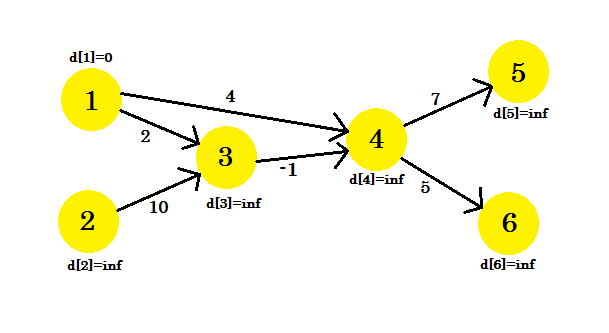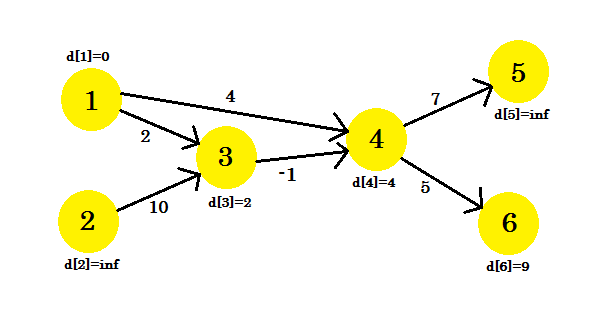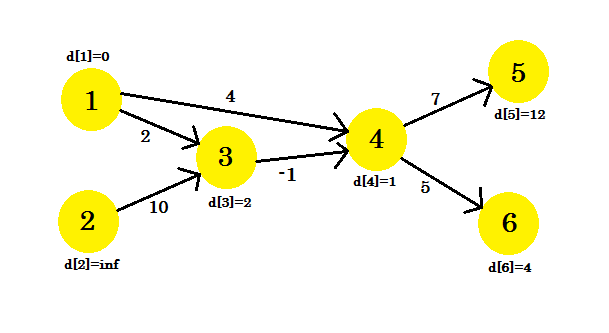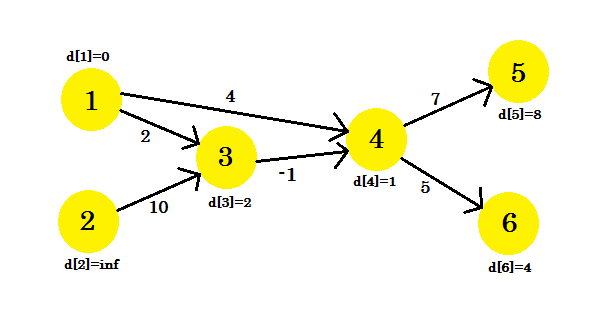# Single Source Shortest Path Algorithm (Given there is a negative cycle in a graph)

suggest change

Before reading this example, it is required to have a brief idea on edge-relaxation. You can learn it from here

Bellman-Ford Algorithm is computes the shortest paths from a single source vertex to all of the other vertices in a weighted digraph. Even though it is slower than Dijkstra’s Algorithm, it works in the cases when the weight of the edge is negative and it also finds negative weight cycle in the graph. The problem with Dijkstra’s Algorithm is, if there’s a negative cycle, you keep going through the cycle again and again and keep reducing the distance between two vertices.

The idea of this algorithm is to go through all the edges of this graph one-by-one in some random order. It can be any random order. But you must ensure, if u-v (where u and v are two vertices in a graph) is one of your orders, then there must be an edge from u to v. Usually it is taken directly from the order of the input given. Again, any random order will work.

After selecting the order, we will relax the edges according to the relaxation formula. For a given edge u-v going from u to v the relaxation formula is:

``````if distance[u] + cost[u][v] < d[v]
d[v] = d[u] + cost[u][v]``````

That is, if the distance from source to any vertex u + the weight of the edge u-v is less than the distance from source to another vertex v, we update the distance from source to v. We need to relax the edges at most (V-1) times where V is the number of edges in the graph. Why (V-1) you ask? We’ll explain it in another example. Also we are going to keep track of the parent vertex of any vertex, that is when we relax an edge, we will set:

``parent[v] = u``

It means we’ve found another shorter path to reach v via u. We will need this later to print the shortest path from source to the destined vertex.

Let’s look at an example. We have a graph:We have selected 1 as the source vertex. We want to find out the shortest path from the source to all other vertices.

At first, d = 0 because it is the source. And rest are infinity, because we don’t know their distance yet.

We will relax the edges in this sequence:

``````+--------+--------+--------+--------+--------+--------+--------+
| Serial |    1   |    2   |    3   |    4   |    5   |    6   |
+--------+--------+--------+--------+--------+--------+--------+
|  Edge  |  4->5  |  3->4  |  1->3  |  1->4  |  4->6  |  2->3  |
+--------+--------+--------+--------+--------+--------+--------+``````

You can take any sequence you want. If we relax the edges once, what do we get? We get the distance from source to all other vertices of the path that uses at most 1 edge. Now let’s relax the edges and update the values of d[]. We get:

1. d + cost = infinity + 7 = infinity. We can’t update this one.
2. d + cost = infinity. We can’t update this one.
3. d + cost = 0 + 2 = 2 < d. So d = 2. Also parent = 1.
4. d + cost = 4. So d = 4 < d. parent = 1.
5. d + cost = 9. d = 9 < d. parent = 4.
6. d + cost = infinity. We can’t update this one.

We couldn’t update some vertices, because the `d[u] + cost[u][v] < d[v]` condition didn’t match. As we have said before, we found the paths from source to other nodes using maximum 1 edge.Our second iteration will provide us with the path using 2 nodes. We get:

1. d + cost = 12 < d. d = 12. parent = 4.
2. d + cost = 1 < d. d = 1. parent = 3.
3. d remains unchanged.
4. d remains unchanged.
5. d + cost = 6 < d. d = 6. parent = 4.
6. d remains unchanged.

Our graph will look like:Our 3rd iteration will only update vertex 5, where d will be 8. Our graph will look like:After this no matter how many iterations we do, we’ll have the same distances. So we will keep a flag that checks if any update takes place or not. If it doesn’t, we’ll simply break the loop. Our pseudo-code will be:

``````Procedure Bellman-Ford(Graph, source):
n := number of vertices in Graph
for i from 1 to n
d[i] := infinity
parent[i] := NULL
end for
d[source] := 0
for i from 1 to n-1
flag := false
for all edges from (u,v) in Graph
if d[u] + cost[u][v] < d[v]
d[v] := d[u] + cost[u][v]
parent[v] := u
flag := true
end if
end for
if flag == false
break
end for
Return d``````

To keep track of negative cycle, we can modify our code using the procedure described here. Our completed pseudo-code will be:

``````Procedure Bellman-Ford-With-Negative-Cycle-Detection(Graph, source):
n := number of vertices in Graph
for i from 1 to n
d[i] := infinity
parent[i] := NULL
end for
d[source] := 0
for i from 1 to n-1
flag := false
for all edges from (u,v) in Graph
if d[u] + cost[u][v] < d[v]
d[v] := d[u] + cost[u][v]
parent[v] := u
flag := true
end if
end for
if flag == false
break
end for
for all edges from (u,v) in Graph
if d[u] + cost[u][v] < d[v]
Return "Negative Cycle Detected"
end if
end for
Return d``````

Printing Path:

To print the shortest path to a vertex, we’ll iterate back to its parent until we find NULL and then print the vertices. The pseudo-code will be:

``````Procedure PathPrinting(u)
v := parent[u]
if v == NULL
return
PathPrinting(v)
print -> u``````

Complexity:

Since we need to relax the edges maximum (V-1) times, the time complexity of this algorithm will be equal to O(V * E) where E denotes the number of edges, if we use `adjacency list` to represent the graph. However, if `adjacency matrix` is used to represent the graph, time complexity will be O(V^3). Reason is we can iterate through all edges in O(E) time when `adjacency list` is used, but it takes O(V^2) time when `adjacency matrix` is used.